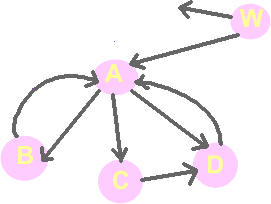# PageRank ComputationsMeaning: The likelihood that a surfer, clicking randomly will arrive at any particular page. Also called the Random Surfer Model: What is the probability that a random surfer will land up on a page?

It is an algorithm used to assign relative importance to nodes of a graph, each of them having a set of inward and outward edges (like the web). PageRank is patented to Stanford University, while Google has the rights to exclusive use. These rights were bought by Google in 2005 for \$336 million.

Essentially, if Page A links to Page B, Google counts it as a "vote'' for Page B.BUT not at votes are equal! How important is the page voting for you? If my webpage has a link to your webpage, that is less important than nytimes.com having a link to your webpage! NYTimes transfers some of its "authority" to you.

How does NYTimes "distribute" its authority among the pages it links to? It just divides its own authority (or PageRank) equally among the pages it links to.

Consider the web site represented by the following graph, representing a set of web pages and the links interconnecting them:## Simplified Algorithm

• ### Algebraic Computation

```PR(A) = PR(W)/2 + PR(B)/1 + PR(D)/1
PR(B) = PR(A)/3
PR(C) = PR(A)/3
PR(D) = PR(A)/3 + PR(C)
PR(W) = 0
```

if we denote PR(X) = x, then the above system of equations can be written as:

```a = w/2 + b + d
b = a/3
c = a/3
d = a/3 + c
w = 0
```

If we try to solve the above system of equations, we see that it has many solutions, all of the form:

```a can be any number
b = a/3
c = a/3
d = 2*a/3
w = 0
```

So, one possible solution is this:

```a = 3
b = 1
c = 1
d = 2
w = 0
```

Such a solution is called "equilibrium" or "fixed point". Because there are many fixed points, it makes sense to care about the relationship among the values of a, b, c, d and w. In other words we can normalize the results to be in a certain range (0 - 7, for example).

If you imagine the page rank as flow of water, originating into the system from the node W above and flowing through the links from one node to the others, the fix point represents the point when the water flow is stable among the nodes/stations of the system.

• ### Iterative computations

• Task 1: We can use MS excel to compute a solution to the above system of equations.
• Task 2: What would happen if node w had only one outgoing link to node A? Set up the system of equations and find its solution(s) using Excel. How can you explain intuitively the result, using the water flow metaphor?

Modify the given graph so that node C is a dead end, i.e. remove the link from node C to node D. Set up the system of equations, use Excel to find a solution, and explain intuitevely what you get with Excel.

## Teleporting Algorithm

We saw that the "non-teleporting" algorithm for computing page-ranks can have problems in certain situations. To avoid these problems, google is using a "teleporting" algorithm for page-rank computations. In this way, 85% of the page-rank for any page is computed as in the non-teleporting way, while the rest 15% of the rank is coming from re-distributing the rank of the system. Intuitevely, in our "water-flow" model, we can think of that second 15% of the rank, as if it was water evaporating from the system, and then coming down to it again, equally in every node, as rain.

In the teporting way, the system of equations for the initial graph, becomes:

PR(A) = 0.85(PR(W)/2 + PR(B)/1 + PR(D)/1) + 0.15/5

PR(B) = 0.85(PR(A)/3) + 0.15/5

PR(C) = 0.85(PR(A)/3) + 0.15/5

PR(D) = 0.85(PR(A)/3 + PR(C)) + 0.15/5

PR(W) = 0.85(PR(W)/2) + 0.15/5

• Task 4: Use Excel to compute the pageRanking of the system above.